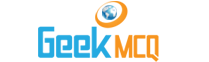Entry Test MCQ :: Work And Energy

11.  Work done by variable force is determine by dividing
 A. force into small interval B. displacement into small interval C. both force and displacement into small intervals D. force into small and displacement into large intervals

12.  Work done on the body equals to the
 A. change in its K.E always B. change in its P.E always C. change in its K.E and change in its P.E D. neither change in K.E and nor change in its P.E

13.  The escape velocity of a body in gravitational field of earth is independent of
 A. its mass B. the angle at which it is thrown C. both its mass and the angle at which it is thrown D. gravitational field of earth

14.  The tides raise the mater in the see roughly in a day
 A. once B. twice C. four time D. eight time

15.  The source of geothermal energy is
 A. decay of radioactive element in the earth B. compression of material in the earth C. residual lost of the earth D. all as said in a - b and c

16.  The highest value of escape velocity in solar system is planet
 A. Earth B. Neptune C. Jupiter D. Moon

17.  Work done by the force of friction is
 A. always positive B. always negative C. positive only for small frictional force D. positive only for large frictional force

18.  Gravitational P.E of a body has
 A. no formula B. a formula mgh only C. a formula D. no general formula

19.  If velocity is doubled then
 A. momentum increases 4 times and K.E increases 2 times B. momentum and K.E. remain same C. momentum increases 2 times and K.E increases constant D. momentum increases 2 times and K.E increases 4 time

20.  When the speed of a moving body is doubled then
 A. its K.E is doubled B. its acceleration is doubled C. its P.E is doubled D. its momentum is doubled